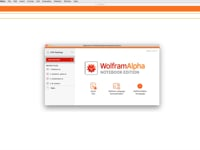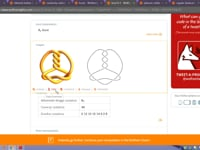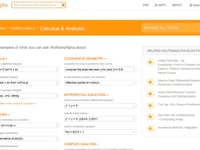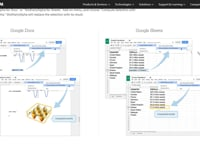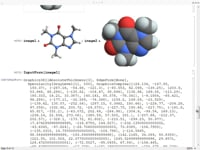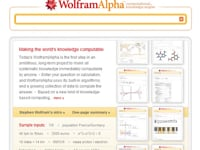Learn how »

# Hands-on Start to Wolfram|Alpha Notebook Edition

Learn the basics of using Wolfram|Alpha Notebook Editionuse free-form input to enter math and science calculations, create interactive notebooks, calculate problems numerically or symbolically and see step-by-step solutions, assign variables and define functions, create 2D and 3D graphics and make them interactive, and turn your notebook into a dynamic presentation. This content requires no prior experience with Wolfram Notebooks or Wolfram|Alpha Notebook Edition.